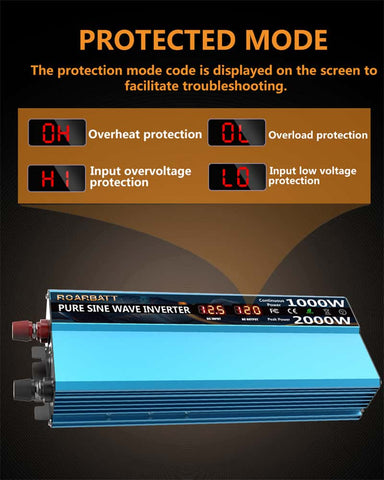# How many amps does a 1000 watt inverter draw?

How many amps does a 1000 watt inverter need to start? Such a question is not very accurate and we need to specify the battery voltage before we know how many amps are needed.Before we can determine the battery voltage, let's understand the operating range of the inverter for the battery voltage. The inverter will have a minimum and maximum voltage that it can operate at. For a 12-volt battery, the minimum voltage is 10.5 volts and the maximum is 15 volts. This means that the inverter will draw more amps at the lower voltage and fewer amps at the higher voltage.

Now that we know the operating range of the inverter, we can determine the battery voltage that is needed. For a 12-volt battery, the minimum voltage is 10.5 volts and the maximum is 15 volts. This means that the inverter will need at least 10.5 volts to start and can operate at up to 15 volts.12VDC 1000W inverter: 10V-15.5V

24VDC 1000W inverter: 20V-31V

48VDC 1000W inverter: 40V-62V

Different battery voltages require different currents. Let's take 12VDC 1000W as an example, in the state of 10VDC, the calculation of 1000W inverter works as follows:

output power / battery voltage = current, i.e. I=P/U: 100A="1000W/10V",

If you're considering a 1000w inverter, one of the things you'll want to look at is its conversion efficiency. The powerinverter.cc has an inverter conversion efficiency of 90%. That means that, at full power, it will require 100Amp/0.9=111.1A from your battery.To be precise, in a 10VDC state, the maximum current required for a 1000W inverter is 111.1A. However, when the battery voltage is at 15.5V, 1000W/15.5V=64.5A, the conversion efficiency is still 90%, and the current at the battery end is only 64.5A/0.88=71.6A.

So, if you're looking for a high-powered inverter with excellent conversion efficiency, the Inverter for powerinverter.cc is worth considering.

Check out our selection of Inverters in our shop to see which ones meet your power needs. For more information, you can check these articles: